Displays the shaded map in 3D with the rgl package.

plot_3d(
heightmap,
zscale = 1,
baseshape = "rectangle",
solid = TRUE,
soliddepth = "auto",
solidcolor = "grey20",
solidlinecolor = "grey30",
water = FALSE,
waterdepth = 0,
watercolor = "dodgerblue",
wateralpha = 0.5,
waterlinecolor = NULL,
waterlinealpha = 1,
linewidth = 2,
lineantialias = FALSE,
theta = 45,
phi = 45,
fov = 0,
zoom = 1,
background = "white",
windowsize = 600,
precomputed_normals = NULL,
asp = 1,
triangulate = FALSE,
max_error = 0,
max_tri = 0,
verbose = FALSE,
...
)

## Arguments

hillshade Hillshade/image to be added to 3D surface map. A two-dimensional matrix, where each entry in the matrix is the elevation at that point. All points are assumed to be evenly spaced. Default 1. The ratio between the x and y spacing (which are assumed to be equal) and the z axis. For example, if the elevation levels are in units of 1 meter and the grid values are separated by 10 meters, zscale would be 10. Adjust the zscale down to exaggerate elevation features. Default rectangle. Shape of the base. Options are c("rectangle","circle","hex"). Default TRUE. If FALSE, just the surface is rendered. Default auto, which sets it to the lowest elevation in the matrix minus one unit (scaled by zscale). Depth of the solid base. Default grey20. Base color. Default grey30. Base edge line color. Default TRUE. If FALSE, no shadow is rendered. Default auto, which sets it to soliddepth - soliddepth/10. Depth of the shadow layer. Default grey50. Color of the shadow. Default auto, which sizes it to 1/10th the smallest dimension of heightmap. Width of the shadow in units of the matrix. Default FALSE. If TRUE, a water layer is rendered. Default 0. Water level. Default lightblue. Color of the water. Default 0.5. Water transparency. Default NULL. Color of the lines around the edges of the water layer. Default 1. Water line tranparency. Default 2. Width of the edge lines in the scene. Default FALSE. Whether to anti-alias the lines in the scene. Default 45. Rotation around z-axis. Default 45. Azimuth angle. Default 0--isometric. Field-of-view angle. Default 1. Zoom factor. Default grey10. Color of the background. Default 600. Position, width, and height of the rgl device displaying the plot. If a single number, viewport will be a square and located in upper left corner. If two numbers, (e.g. c(600,800)), user will specify width and height separately. If four numbers (e.g. c(200,0,600,800)), the first two coordinates specify the location of the x-y coordinates of the bottom-left corner of the viewport on the screen, and the next two (or one, if square) specify the window size. NOTE: The absolute positioning of the window does not currently work on macOS (tested on Mojave), but the size can still be specified. Default NULL. Takes the output of calculate_normals() to save computing normals internally. Default 1. Aspect ratio of the resulting plot. Use asp = 1/cospi(mean_latitude/180) to rescale lat/long at higher latitudes to the correct the aspect ratio. Default FALSE. Reduce the size of the 3D model by triangulating the height map. Set this to TRUE if generating the model is slow, or moving it is choppy. Will also reduce the size of 3D models saved to disk. Default 0.001. Maximum allowable error when triangulating the height map, when triangulate = TRUE. Increase this if you encounter problems with 3D performance, want to decrease render time with render_highquality(), or need to save a smaller 3D OBJ file to disk with save_obj(), Default 0, which turns this setting off and uses max_error. Maximum number of triangles allowed with triangulating the height map, when triangulate = TRUE. Increase this if you encounter problems with 3D performance, want to decrease render time with render_highquality(), or need to save a smaller 3D OBJ file to disk with save_obj(), Default TRUE, if interactive(). Prints information about the mesh triangulation if triangulate = TRUE. Additional arguments to pass to the rgl::par3d function.

## Examples

#Plotting a spherical texture map of the built-in montereybay dataset.
# \donttest{
montereybay %>%
plot_3d(montereybay,zscale=50)
render_snapshot(clear = TRUE)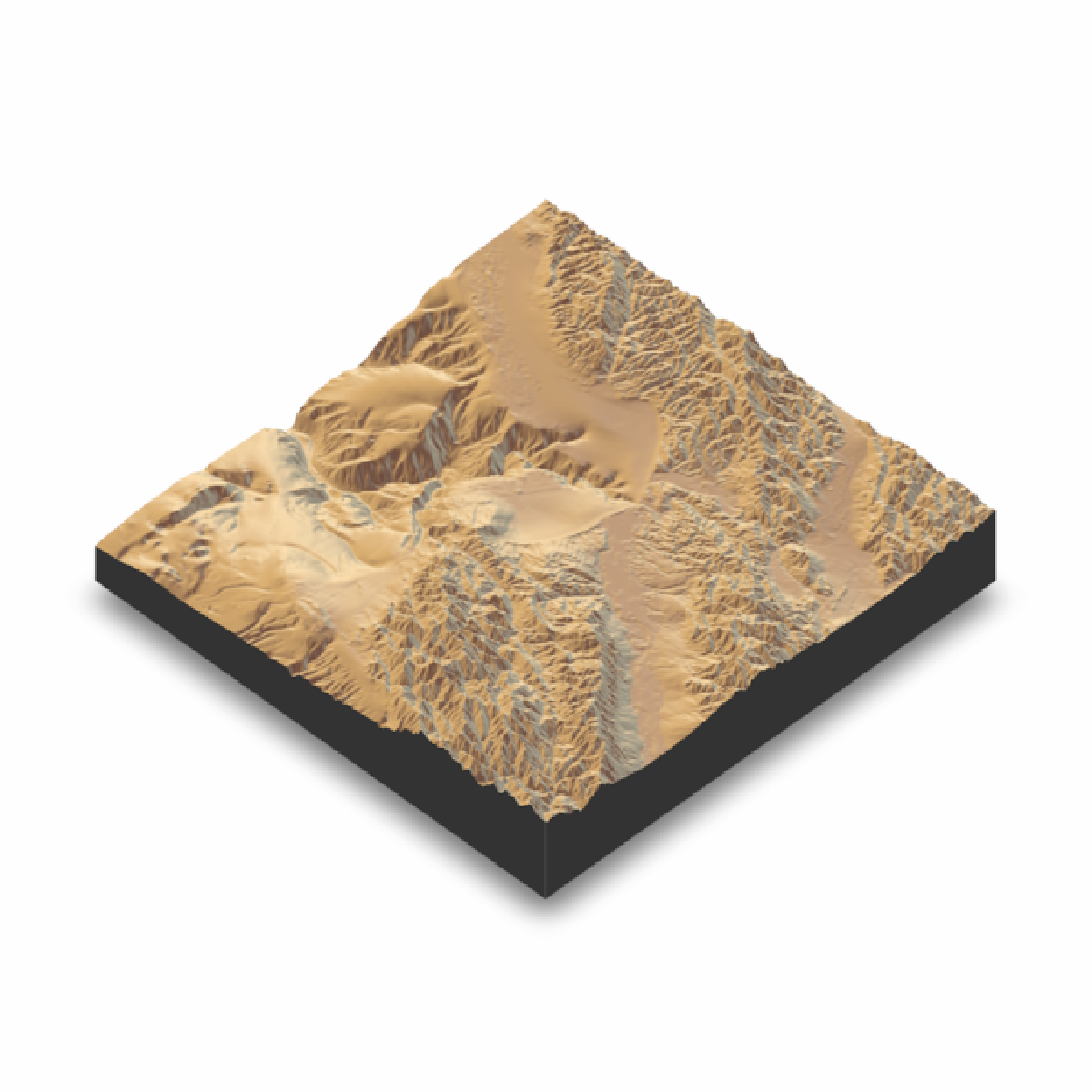# }

#With a water layer
# \donttest{
montereybay %>%
plot_3d(montereybay, zscale=50, water = TRUE, watercolor="imhof2",
waterlinecolor="white", waterlinealpha=0.5)
render_snapshot(clear = TRUE)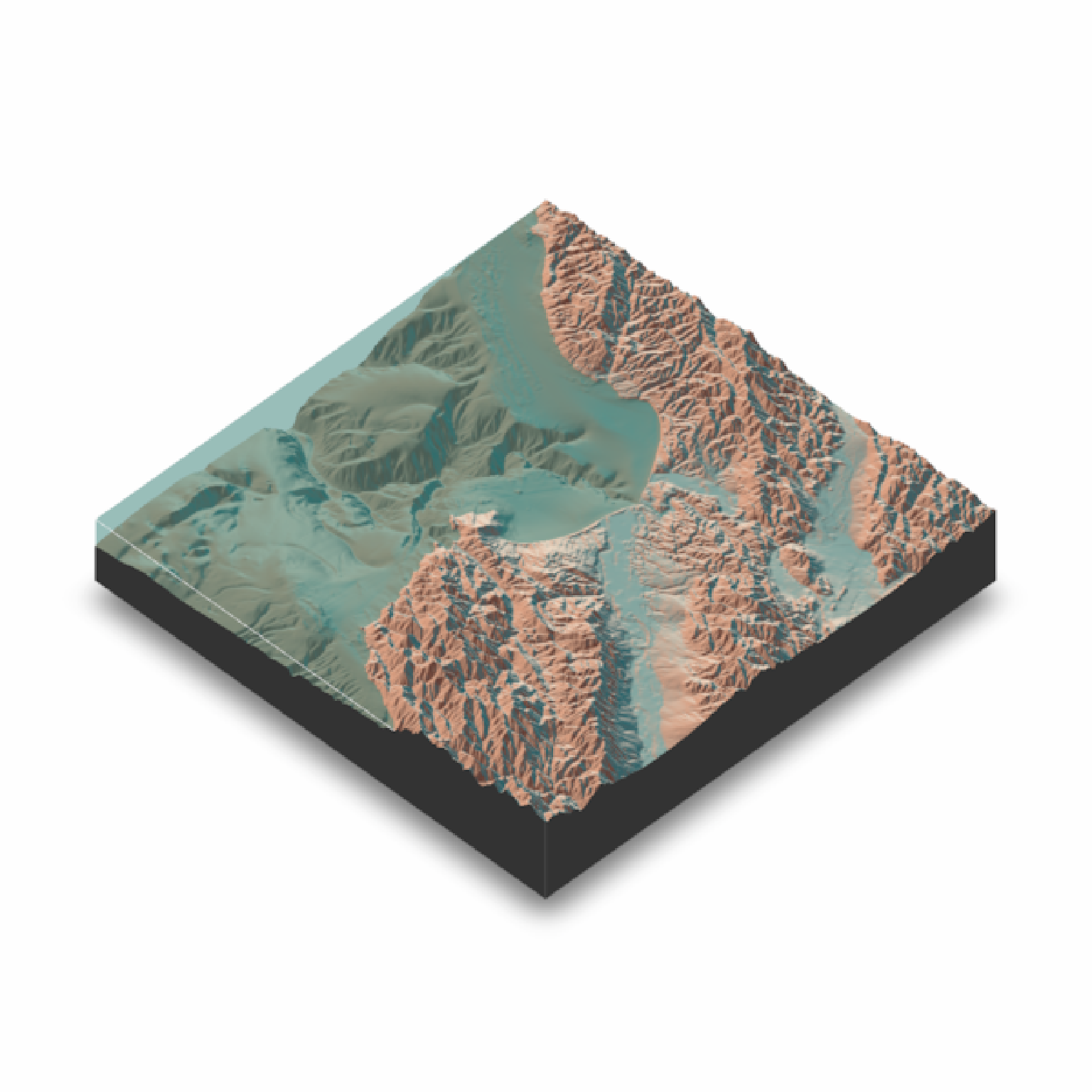# }

#We can also change the base by setting "baseshape" to "hex" or "circle"
# \donttest{
montereybay %>%
plot_3d(montereybay, zscale=50, water = TRUE, watercolor="imhof1", theta=-45, zoom=0.7,
waterlinecolor="white", waterlinealpha=0.5,baseshape="circle")
render_snapshot(clear = TRUE)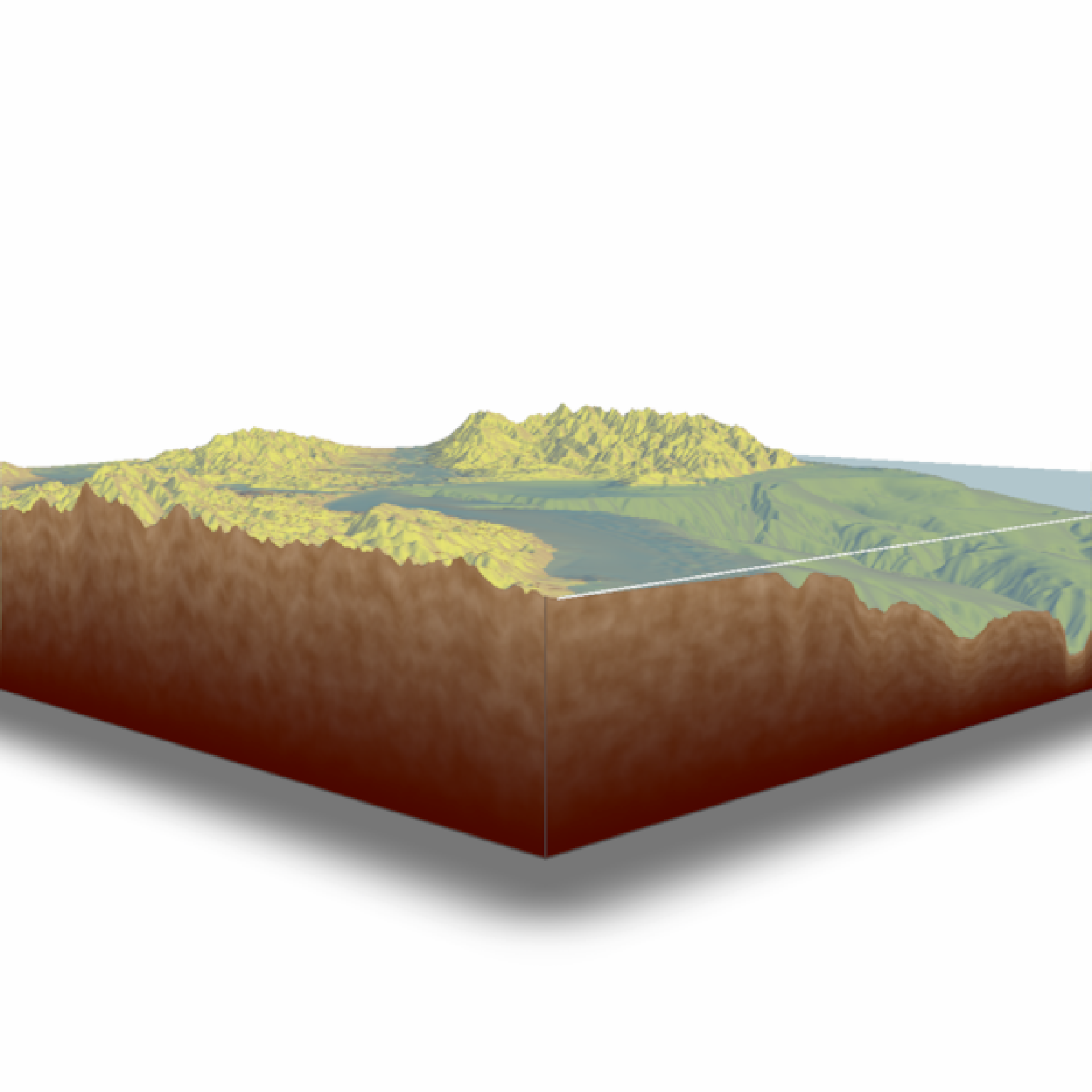# }

# \donttest{
montereybay %>%
plot_3d(montereybay, zscale=50, water = TRUE, watercolor="imhof1", theta=-45, zoom=0.7,
waterlinecolor="white", waterlinealpha=0.5,baseshape="hex")
render_snapshot(clear = TRUE)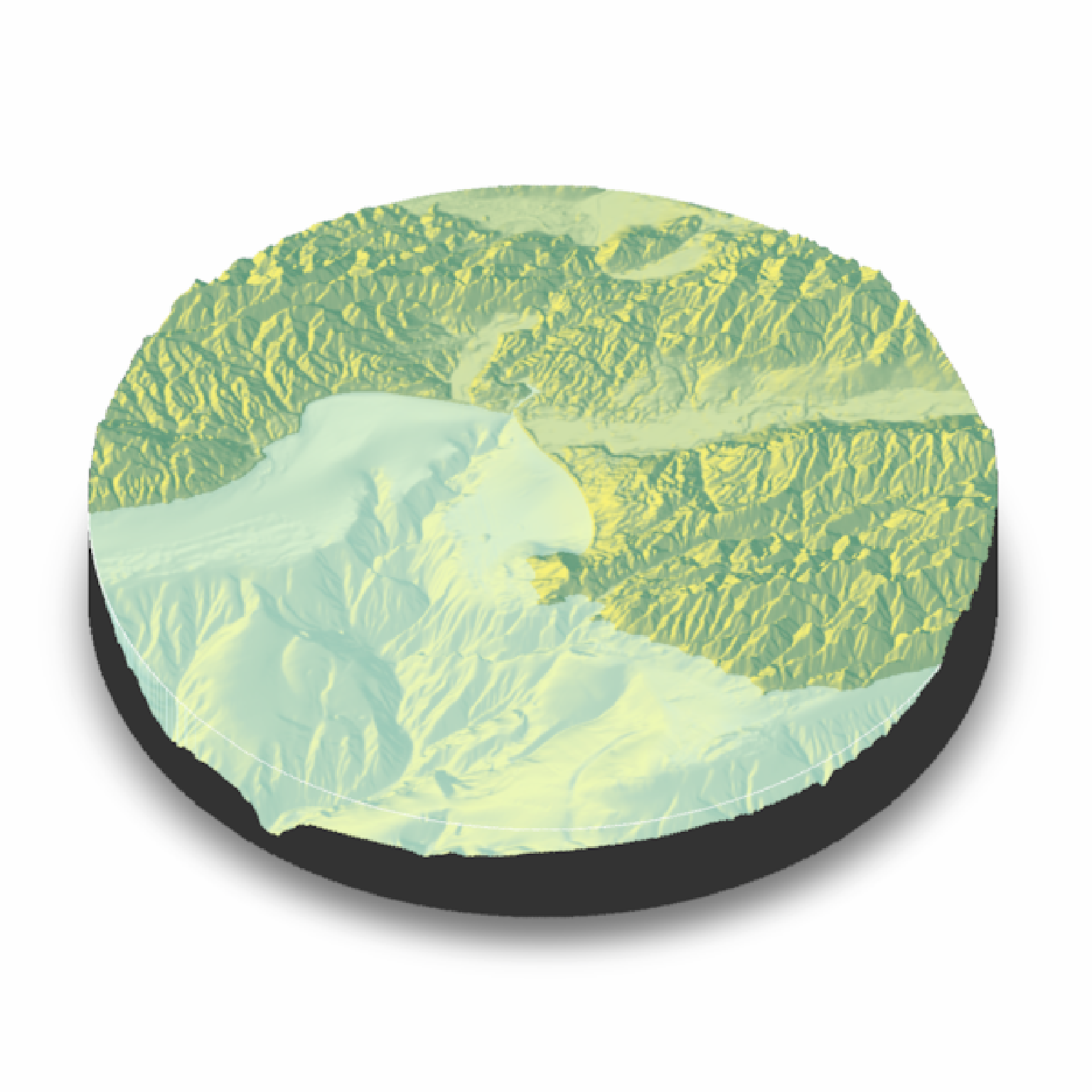# }

#Or we can carve out the region of interest ourselves, by setting those entries to NA
#to the elevation map passed into plot_3d

#Here, we only include the deep bathymetry data by setting all points greater than -10
#in the copied elevation matrix to NA.

mb_water = montereybay
mb_water[mb_water > -10] = NA

# \donttest{
montereybay %>%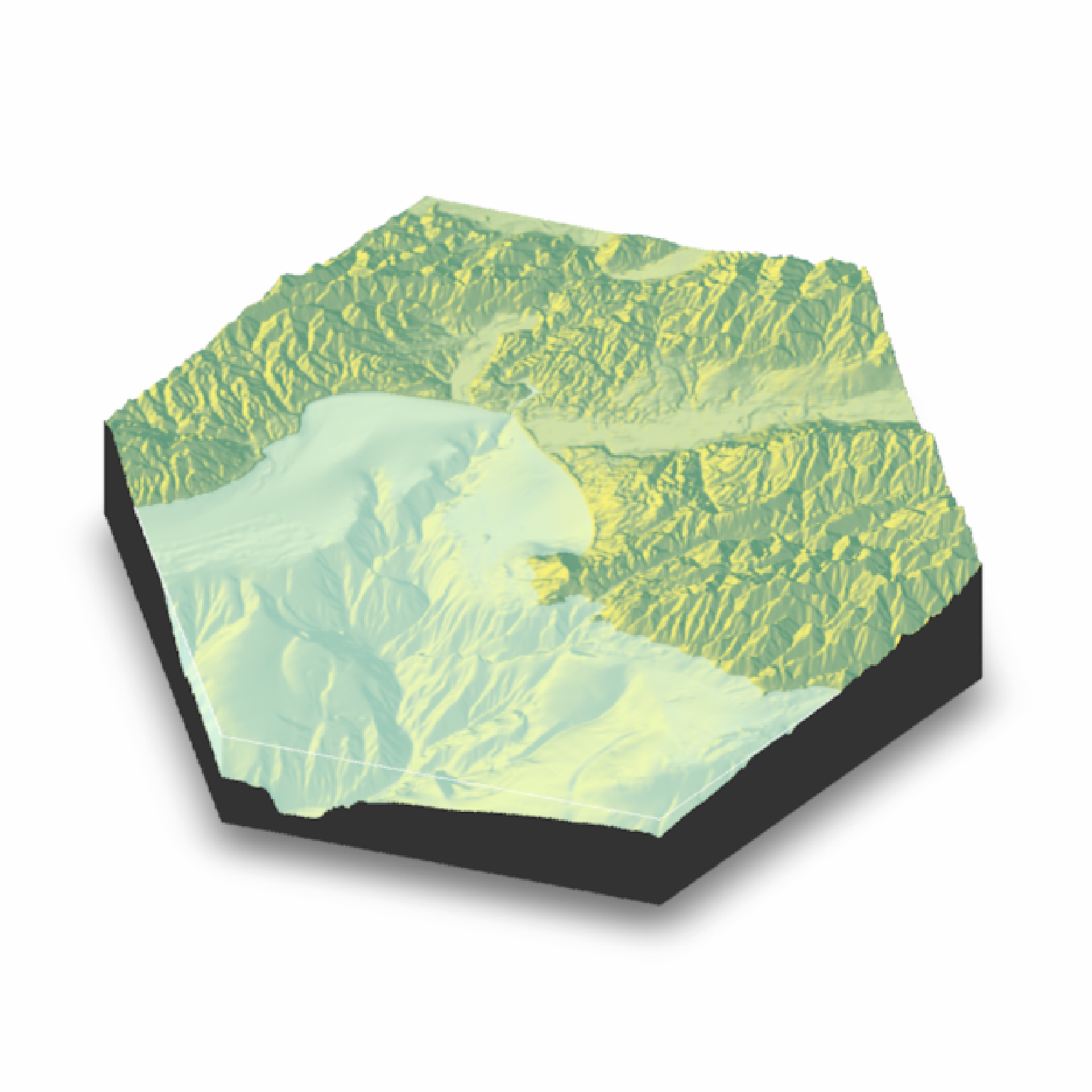# }